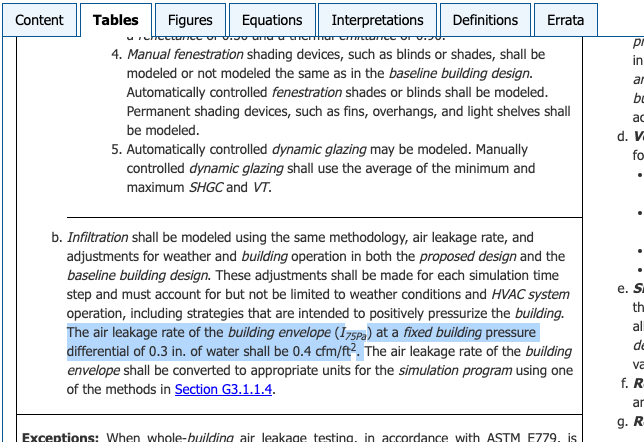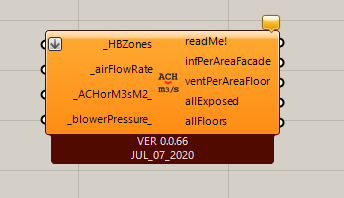# ASHRAE 90.1 Infiltration Air Leakage Input?

Hi all,

I have a question regarding the right inputs for ASHRAE 90.1 Appendix G modeling related to air infiltration? Appendix G Table G3.1 Modeling Requirements for Calculating Proposed and Baseline Building Performance, section 5(b) specifies an input of 0.4 cfm/ft2 (0.0203 m3-s/m2) @ 75Pa (0.3 in-water):so I’m wonder how to enter that correctly in the Honeybee ApplyLoads component? That component is looking for input values at 4Pa, right? So is it ok to just use linear interpolation to convert 0.4cfm/ft2 @75 down to @4 ? So something like:

Does that seem correct, given the input units that this component is looking for? I get a value of 0.00018 m3-s/m2 envelope area.

Appreciate any thoughts of input if that seems right, or if there is another approach that folks know of to do this correctly?

thanks!
@edpmay

@edpmay

Airflow scales roughly according to the square root of the pressure difference across an opening (from Bernoulli’s principle), so you can’t treat it linearly.

For infiltration calculations, the 4 Pa assumption comes from the Sherman and Grimsrud method, which takes a series of airflow measurements from building depressurization tests at high (i.e 75) pressure and fits the data to predict airflow at 4 Pa (typical building envelope pressure).

The formula for infiltration they use is:

\dot{q}_{inf} = C \Delta{P}^n

where:
P = Pressure [Pa]
\dot{q}_{inf} = airflow per exterior facade area [\frac{m^3}{m^2 s}]

C and n are coefficients for the regression, and are also unknown. You’ll have to make an assumption for one of them. A value of 0.65 for n is typically used. With that you can solve for the C value used in the 75 Pa test and use that value to calculate the 4 Pa airflow, like this:

\begin{align} C_{4_{PA}} &= \frac{\dot{q}_{inf}}{\Delta{P}^n} \\ &= \frac{0.0203}{75^{0.65}} \\ &= 0.0012266 \end{align}

Using that I get:

\begin{align} \dot{q}_{inf} &= 0.0012266 \cdot 4^{0.65} \\ &= 0.00302 \text{ } \frac{m^3}{m^2 s} \end{align}

The one last thing I’ll add is that it’s a little nicer to take the log of all these values first, and do your derivations that way, because the log scales all the values to similar precision and for that reason preserves accuracy a little better. In the non-log version you end up mixing high and low values and that causes some precision loss. But that’s a detail and the above method will work fine.

EDIT
I double checked your initial conversion because 0.00302 seemed too leaky. I’m getting 0.4 cfm/ft2 = 0.002032 m3/m2-s from google, if we substitute that the airflow rate is 0.000302 which seems more reasonable.

2 Likes

Thats super helpful! Thanks so much. Really appreciate it.

on a side note: didn’t there used to be a component for doing this as part of the ‘old’ Honeybee? Is there no such component for doing this conversion anymore? I was rummaging around on the ‘new’ HB components but didn’t see one? Or did I just miss it?

Regardless, this is super clear and easy to implement now. thank you so much!!
@edpmay

@edpmay no problem. I don’t recall a legacy component to do this, but I could be wrong, I don’t think I know all the components in LB.

Thanks for the thorough response on this one, @SaeranVasanthakumar .

Yes, there was a legacy component that did this (along with some other things) and I didn’t put it in the LBT plugin yet since I originally wasn’t sure how I was going to implement ACH-based infiltration.Now that the ACH-based workflows were implemented through the “HB Apply Absolute Load Values”, I will add another component that’s specifically for the blower-pressure conversion. I can do this quickly now and I will post back once the component is merged.

The component has been added to the LBT plugin and you can see that we are making use of your staticmethod in the core libraries, @SaeranVasanthakumar .

Now, this is all that you need to do to setup infiltration according to the ASHRAE 90.1 specification there:

1 Like

Good code re-use! I forgot that I already wrote that Cq conversion.

Thanks for reminding this Legacy one. I miss from it the possibility to define infiltration as ACH.
What are the chances of bringing this to LBT?

Thanks,
-A.

Fantastic! Thanks so much @chris - this is great.

One quick question: I get a slightly different result from this component than the method described by @SaeranVasanthakumar above in this thread?

Seems like the C_qa is calculating differently? I understood from the description above that C_qa is just infiltration / (pressure ^ n) ? Not a huge difference. I guess its just that you are including the density of air in your method?

?

am I understanding that right?

regardless - thanks!!
@edpmay

@edpmay

Good catch. I (confusingly) am referring to two slightly different values with the same name which is leading to a subtle bug here.

The formula I shared above assumes air density is constant, so I ignored it’s impact on the Cq coefficient, whereas the Ladybug method is solving Cq for an EnergyPlus simulation where density is evolved per timestep, and thus incorporates a density assumption. The relationship is like this:

C_{LB} = \frac{C_{constant_{\rho}}}{\rho}
C_{LB} = mass flow coefficient [kg/m2-s]
C_{constant_{\rho}} = volume flow coefficient [m3/m2-s]

If you plug in a density assumption into the formula I shared above, you can see that the density cancels out during the conversion, which is why I ignored it.

The Ladybug staticmethod will add a density value by default if it’s not specified, which is where we’re getting the bug. It’s therefore returning a mass flow coefficient instead of a volume flow coefficent. @chris the simplest way to solve this is just to specifiy an air_density argument of 1:

C_qa = RoomEnergyProperties.solve_norm_area_flow_coefficient(
_infilt_per_exterior, delta_pressure=_blower_pressure_,
air_density=1)


And then change the docstring for C_qa to “volume”:

C_qa: Air volume flow coefficient per square meter at 1 Pa [m3/m2/s/P^n].


Then the values from my calculation (x0.1) above and Ladybug will match.

Thanks for finding the bug @edpmay and for proposing a solution, @SaeranVasanthakumar . I just merged the fix:

The infiltration ACH capability is already supported by another component in LBT:

Thanks @chris,
Yes, I am aware of the AbsoluteLoadVals component. The thing is that i’m in the process of defining a (full) program set, so i know that i can “forget” about loads definitions later on. That’s why i wrote the above and still think that it can be useful and more consistent with the idea of the sets. Hope this makes sense.
This is not critical but yet useful.
Thanks,
-A.

Ah, I got confused because you said you missed a feature of Legacy but there were no program objects in Legacy. So you are talking about adding a new feature of an ACH property on program infiltration. The main reason why I want to keep the infiltration assignment by ACH on a room-by-room basis is that converting this ACH to m3/m2-s and having only one infiltration property to check per room makes it much easier to figure out what infiltration rate is assigned and QA/QC a model. I can see that the ACH input for the minimum ventilation requirement already creates a significant amount of confusion as you can see in discussions like this. So I really don’t want to add another property that people will have to check unless it makes other common workflows REALLY convenient. So I would ask, is the assignment of infiltration using the “Absolute Load” component really that inconvenient as to merit making the QA/QC workflows more complicated?

Thanks @chris!
Making things complicated is the last thing i want …The 3 options in the infiltration program are good enough to use the m2/m2 method. Though ACH is much easier to explain.

-A.

2 Likes